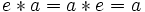# Binary operation on magma determines neutral element

Suppose$(S,*)$ is a magma (set$S$ with binary operation$*$). Then, if there exists a neutral element for$*$ (i.e., an element$e$ such that$e * a = a * e = a$ for all$a \in S$), the element$e$ is uniquely determined by$*$.
In the case that$*$ is associative, this says that the identity element (neutral element) of a monoid is completely determined by the binary operation. This yields the fact that monoids form a non-full subcategory of semigroups.